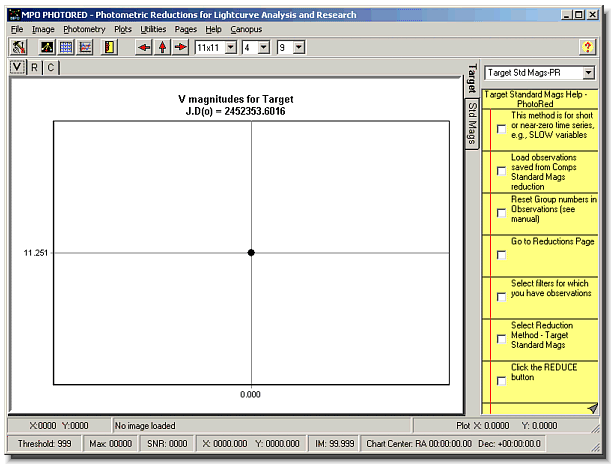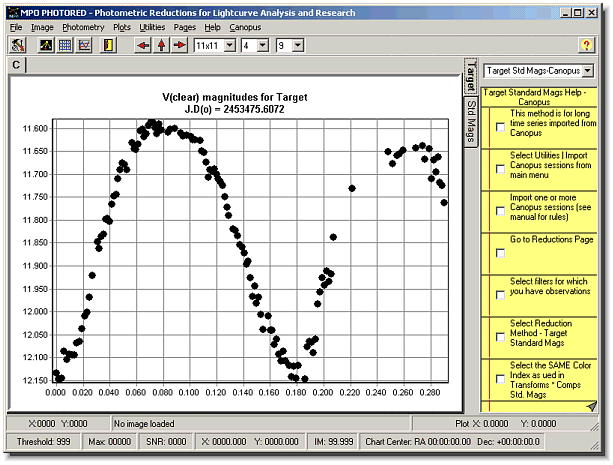# The Minor Planet Observer andPalmer Divide Observatory

(opens new window)

MPO Support

CALL web site

Society for Astronomical Sciences

99999999999999999999

Home
Up

# PhotoRed Reductions Routines - Target Standard MagnitudesUsing data created in PhotoRed

Using data imported from CanopusThis routine is similar to the one for comparison stars save that the results are handled a bit differently. For one, the values are not stored in the Transforms form. Another is that in addition to the plots you see above, if the data was imported from a Canopus session, it can be exported out to files that can be used in Canopus for analysis or merging with other Canopus data.

As with the comparisons routine, you need to have observations in only one filter but you must know the standard color index of the target before you run this routine. If the asteroid is a target, you should shoot three images in each filter in as short a time as possible. It’s best not to group images by filter when shooting but in sequence, e.g., VRVRVR and not VVVRRR. The reason is so that when you measure the images, you put the first V and R in group 1, the next V and R in group 2, and so on. This keeps the two different filter images close together in time. PhotoRed then finds the average for the three readings to provide the single color index value. Were you to shoot in VVVRRR fashion and the asteroid is changing magnitude quickly (as in the second plot above), then the color index value is affected. For example, if the asteroid is fading, then the average of the three R images will be dimmer more than it should be and so the result V-R greater than the real value.

Why the two plots for the same routine? It depends on the data you provide. The first plot (partly covered with only one data point) was the result of measuring a target field in PhotoRed in order to get a single V reading for the target, i.e., there was no time-series involved. This might be the case if you’re working a set of variables and getting only one or very small number of readings per night. The second plot is the result of working with data that was imported from Canopus where a protracted time-series session was created to monitor an asteroid (an eclipsing variable star might have a similar curve). As an aside, note the vertical axis is divided into 0.05m steps. This was high quality data with errors generally <0.01m.

The process for this routine follows these steps

1. For each group, PhotoRed finds the average value of the instrumental magnitudes for each comparison and the target.
2. Using the transforms and standard color indices for the target and each comparison, PhotoRed uses the differential formula to find the reduced standard differential magnitude of the target based on one comparison at a time.
3. Mathematically

`DMf = (TIavg – CIxavg) + Tf * (CIT – CIxc)`
```Where 	DMf 	differential standard magnitude in filter f
TIavg 	average of target instrumental magnitudes
CIxavg 	average of comparison X instrumental magnitudes
Tf 	transform for filter f
CIt 	standard color index of target
CIxc 	standard color index of the comparison X ```
4. The average of the reduced differential values is then applied to the average of the reduced average standard magnitudes of the comparisons to find the final standard magnitude of the target for the group.

Mathematically

`SMf = Avg(MCf) + Avg(DMf1-n)`
```Where 	SMf 		standard magnitude of target in filter f
Avg(Mcf) 	average of reduced standard magnitudes of the comparisons
Avg(DMf1-n) 	average of standard differentials 1 through N```

It’s important to appreciate what’s going on in step 2. Say five comparisons are used. One way to find the target’s standard magnitude might be to find the average of the reduced standard magnitudes of the comparisons and simply subtract that from the reduced standard magnitude of the target. That would work if all the comparisons and target were exactly the same color. Since they most likely are not, the standard color index for each comparison and the target comes into play to get the best possible standard magnitude for the target. To do that without the differential approach would require having observations in two standard filters. Instead, using the transforms based on one-color observations and known standard color indices, a reduced differential standard magnitude of the target versus a comparison is found for each comparison, giving five differential values.

Step 3 takes the average of those differential values and applies them to the average of the standard magnitudes of the comparisons to get the final standard magnitude of the target.

 This site is best viewed at 1024x768 and higher This page was last updated on 01/19/11 05:14 -0700. All contents copyright (c) 2005-2011, Brian D. Warner Trademarks are the property of their respective owners